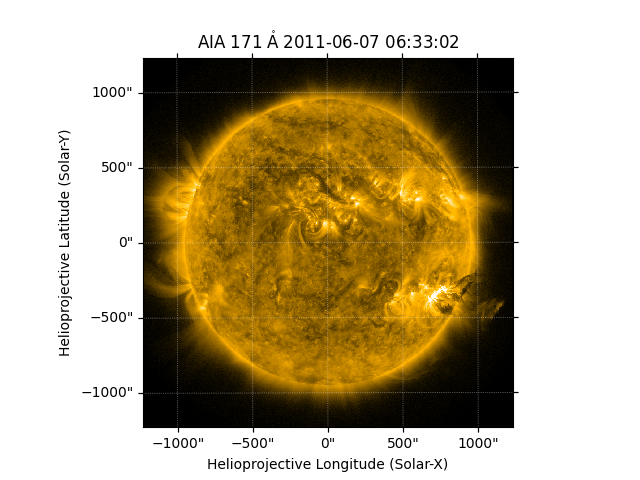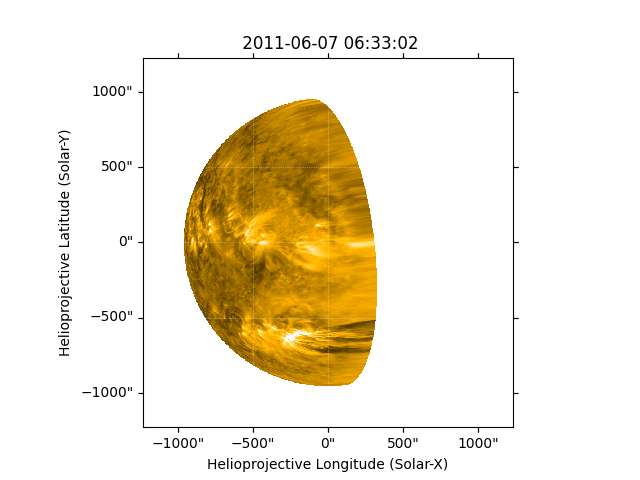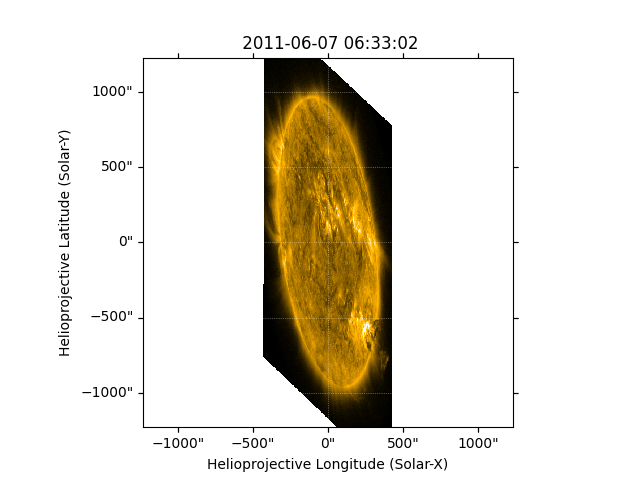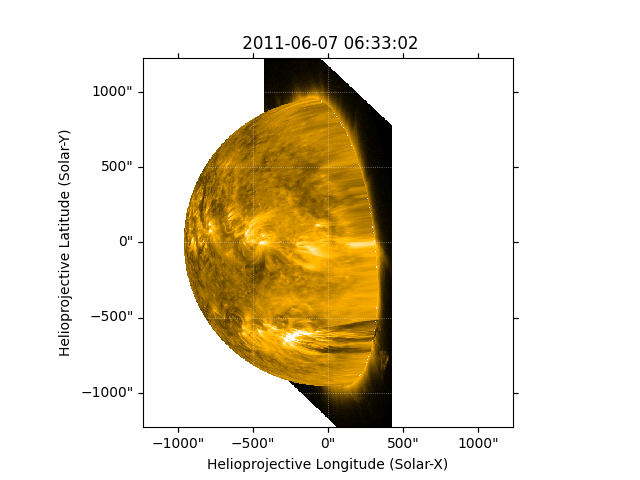# Reprojecting Using a Spherical Screen#

This example demonstrates how you can reproject an image as if it lies on the inside of a spherical screen and the observer is not at the center of the sphere. This functionality is primarily for visualization purposes, since features in the image are unlikely to actually lie on this spherical screen.

You will need reproject v0.6 or higher installed.

import matplotlib.pyplot as plt

import astropy.units as u
from astropy.coordinates import SkyCoord

import sunpy.map
from sunpy.coordinates import Helioprojective
from sunpy.data.sample import AIA_171_IMAGE


We will use one of the AIA images from the sample data. We fix the range of values for the Map’s normalizer.

aia_map = sunpy.map.Map(AIA_171_IMAGE)
aia_map.plot_settings['norm'].vmin = 0
aia_map.plot_settings['norm'].vmax = 10000

fig = plt.figure()
aia_map.plot(axes=ax)
plt.show()Let’s define a new observer that is well separated from Earth.

new_observer = SkyCoord(70*u.deg, 20*u.deg, 1*u.AU, obstime=aia_map.date,
frame='heliographic_stonyhurst')


Create a WCS header for this new observer using helioprojective coordinates.

out_shape = aia_map.data.shape

out_ref_coord = SkyCoord(0*u.arcsec, 0*u.arcsec, obstime=new_observer.obstime,
frame='helioprojective', observer=new_observer,
rsun=aia_map.coordinate_frame.rsun)
out_shape,
out_ref_coord,
scale=u.Quantity(aia_map.scale),
instrument=aia_map.instrument,
wavelength=aia_map.wavelength
)


If you reproject the AIA Map to the perspective of the new observer, the default assumption is that the image lies on the surface of the Sun. However, the parts of the image beyond the solar disk cannot be mapped to the surface of the Sun, and thus do not show up in the output.

outmap_default = aia_map.reproject_to(out_header)

fig = plt.figure()
outmap_default.plot(axes=ax)
plt.show()You can use the different assumption that the image lies on the surface of a spherical screen centered at AIA, with a radius equal to the Sun-AIA distance. The curvature of the spherical screen is not obvious in this plot due to the relatively small field of view of AIA (compared to, say, a coronagraph).

with Helioprojective.assume_spherical_screen(aia_map.observer_coordinate):

fig = plt.figure()
outmap_screen_all.plot(axes=ax)
plt.show()Finally, you can specify that the spherical-screen assumption should be used for only off-disk parts of the image, and continue to map on-disk parts of the image to the surface of the Sun.

with Helioprojective.assume_spherical_screen(aia_map.observer_coordinate,
only_off_disk=True):